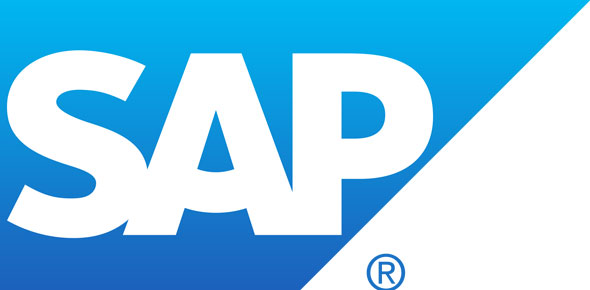# 3.2 SAP S/4HANA For Management Accounting Associates Certification

19 Questions | Total Attempts: 155SettingsCreate your own QuizSAP S/4HANA for Management Accounting Associates Certification

• 1.
For which revenue carrying cost objects does the system perform results analysis? There are 3 correct answers to this question. 3.2
• A.

Cost center

• B.

Internal order

• C.

Work breakdown structure

• D.

• E.

Customer service orders

• 2.
Which object do you configure to reuse material cost estimate for the costing process? 3.2
• A.

Group costing

• B.

Transfer control

• C.

Valuation variant

• D.

Quantity structure control

• 3.
What can you analyze in detail when you transfer the cost component split from product cost planning to costing-based CO-PA? 3.2
• A.

Variance categories

• B.

Cost of goods manufactured

• C.

Cost of goods sold

• D.

Sales revenues

• 4.
You want to use a template for activity and process assignment to your material cost estimate. What do you need to do?* 3.2
• A.

Use the costing sheet of the costing view 1 in the template determination table

• B.

Use the template allocation function in the material cost estimate and the costing run

• C.

Assign a template to an operation in the routing of the material

• D.

Assign a template directly to costing view 1 of the material master record

• 5.
Which parameters can you enter when you create a costing run? There are THREE correct answers for this question 3.2
• A.

Costing variant

• B.

Valuation variant

• C.

Costing version

• D.

Transfer control

• E.

Production version

• 6.
Which object can you configure t offset overhead surcharges for a cost object? There are THREE correct answers for this question 3.2
• A.

Internal order

• B.

Profit center

• C.

• D.

Profitability segment

• E.

Cost center

• 7.
What is required before you can create a multi-level material cost estimate without quantity structure? 3.2
• A.

Material master with costing view

• B.

Material master with accounting view and with MRP views

• C.

Specific costing variants for material costings without quantity structure

• D.

Specific valuation variants for material costings without quantity structure

• 8.
Which cost estimates must you reuse with the transfer control functionality? 3.2
• A.

Cost estimates within the same plant

• B.

Cost estimates with same cost component structure

• C.

Cost estimates across company codes

• D.

Cost estimates with quantity structure

• 9.
Which process determines the target costs of the cost object in a product cost by order scenario? 3.2
• A.

Actual costs calculation

• B.

Work in progress (WIP) calculation

• C.

Variance calculation

• D.

Accrual calculation

• 10.
Which views can you use to analyze the costing results within the costing run tool? There are THREE correct answers for this question 3.2
• A.

Costing levels report

• B.

Variance report

• C.

Material list report

• D.

Analysis report

• E.

Work in progress report

• 11.
In a make-to-order scenario, you use a sales order with an assigned production order and non-valuated sales order stock. Which process do you use to post actual costs on a sales order in SAP S/4HANA? There are TWO correct answers for this question 3.2
• A.

Delivery from production order to sales order stock

• B.

Production order settlement

• C.

External goods receipt on sales order stock

• D.

Production order confirmation

• 12.
Independent from the cost object, which parameters are always contained in a costing variant? There are TWO correct answers for this question 3.2
• A.

Reference variant

• B.

Valuation variant

• C.

Transfer control

• D.

Costing type

• 13.
What do you use to map the primary cost component split to the production cost component structure? 3.2
• A.

Allocation structure

• B.

Assignment scheme

• C.

Cost Component groups

• D.

Transfer structure

• 14.
What do you get if you mark a standard price cost estimate? There are TWO correct answers for this question 3.2
• A.

An accounting document for material ledger

• B.

A future standard price

• C.

A new status in the cost estimate

• D.

A cost-based CO-PA document for material revaluation

• 15.
What can you use to difference two or more cost estimates for the same material? There are TWO correct answers for this question 3.2
• A.

Costing version

• B.

Costing variant

• C.

Transfer control

• D.

Valuation variant

• 16.
What do you do to create a primary cost component split? There are TWO correct answers for this question 3.2
• A.

Assign a cost component structure to the CO-version

• B.

Use the cost splitting tool on cost centers

• C.

Run an activity price calculation

• D.

Enter split activity prices manually

• 17.
Which processes belong to the “final costing” of cost objects? There are TWO correct answers for this question 3.2
• A.

Revaluation at actual costs

• B.

Work in process

• C.

Variance calculation

• D.

• 18.
For which objects can you revaluate activities and business processes at actual prices? There are THREE correct answers for this question 3.2
• A.

Profit centers

• B.

Work breakdown structure elements

• C.

Work centers

• D.

Cost centers

• E.

Profitability segments

• 19.
Which object do you have to assign the cost component structure to? 3.2
• A.

Valuation variant

• B.

Costing variant

• C.

Material master record

• D.

Costing type

Related TopicsBack to top
×

Wait!
Here's an interesting quiz for you.